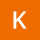Home
IT Knowledge
Inspiration
Languages
EN

# Python - find text in string

0 points
Created by:Keisha-Acosta
260

In this article, we would like to show you how to find text in string in Python.

Quick solution:

``string.find(value, start, end)``

Where:

• `value` - the substring to search for,
• `start` - specifies where to start the search (default value is 0),
• `end` - specifies where to end the search (default value is the end of the string).

Note:

The `find()` method returns `-1` if the substring wasn't found.

## Practical example

In this example, we use `string.find()` method to find the first occurrence of the specified substring.

``````numbers = "ABCDE"

print(numbers.find("A"))         # 0
print(numbers.find("A", 2, 4))   # -1

print(numbers.find("CD"))        # 2
print(numbers.find("CD", 1, 3))  # -1``````

Output:

``````0
-1
2
-1``````

Note:

The `find()` method is similar to the `index()` method, but the `index()` method raises an exception instead of `-1` when the substring wasn't found.